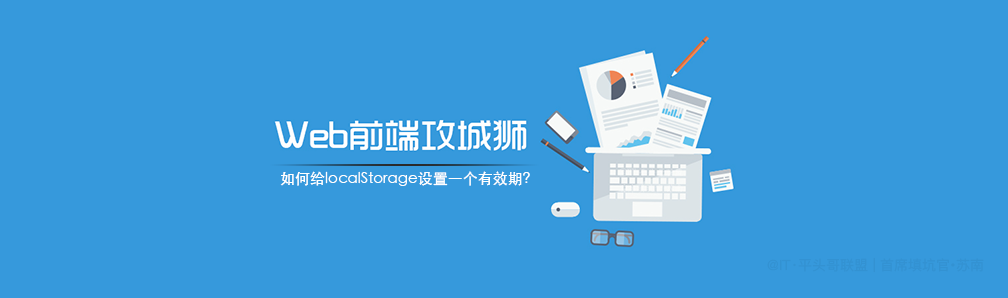## 前言``````Cookie从你设置的时候，就会给个时间，不设置默认会话结束就过期；

``````

## 思路

``````//示例一：
localStorage.setItem('test',1234567);
let test = localStorage.getItem('test');
console.log(typeof test, test);

//示例二：
localStorage['name'] = 'web秀';
console.log(localStorage['name']);
/*

"1234567" ,'web秀',

*/
``````

### 重写 set(存入) 方法：

``````set(key, value, expired) {
/*
* set 存储方法
* @ param {String} 	key 键
* @ param {String} 	value 值，
* @ param {String} 	expired 过期时间，以分钟为单位，非必须
*/
let source = this.source;
source[key] = JSON.stringify(value);
if (expired){
source[`\${key}__expires__`] = Date.now() + 1000*60*expired
};
return value;
}
``````

### 重写 get(获取) 方法

``````get(key) {
/*
* get 获取方法
* @ param {String} 	key 键
* @ param {String} 	expired 存储时为非必须字段，所以有可能取不到，默认为 Date.now+1
*/
const source = this.source,
expired = source[`\${key}__expires__`]||Date.now+1;
const now = Date.now();

if ( now >= expired ) {
this.remove(key);
return;
}
const value = source[key] ? JSON.parse(source[key]) : source[key];
return value;
}
``````

### 重写 remove(删除) 方法

``````remove(key) {
const data = this.source,
value = data[key];
delete data[key];
delete data[`\${key}__expires__`];
return value;
}
``````
``````class storage {

constructor(props) {
this.props = props || {}
this.source = this.props.source || window.localStorage
this.initRun();
}
initRun(){
/*
* set 存储方法
* @ param {String} 	key 键
* @ param {String} 	value 值，存储的值可能是数组/对象，不能直接存储，需要转换 JSON.stringify
* @ param {String} 	expired 过期时间，以分钟为单位
*/
const reg = new RegExp("__expires__");
let data = this.source;
let list = Object.keys(data);
if(list.length > 0){
list.map((key,v)=>{
if( !reg.test(key )){
let now = Date.now();
let expires = data[`\${key}__expires__`]||Date.now+1;
if (now >= expires ) {
this.remove(key);
};
};
return key;
});
};
}
}
``````